Latest Banking jobs   »   Quantitative Aptitude Quiz For SBI/IBPS PO...

# Quantitative Aptitude Quiz For SBI/IBPS PO Mains 2021- 21st December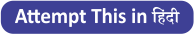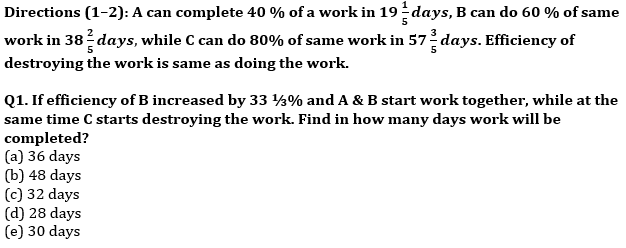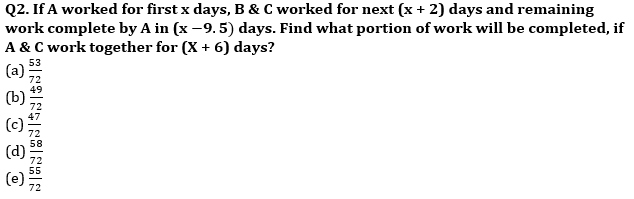Directions (3 – 4): A & B entered into a business by making investment of Rs.7200 and Rs.9600 respectively. After six months A & B both added amount in the ratio of 7: 10. (Business in which A & B entered last for total 12 months)?

Q3. If Satish & Veer invested equal amount as A & B invested for last six months respectively and both Satish and Veer invested for equal time period. Veer got Rs. 25500 as profit share out of total profit of Rs. 44250, then find the amount that A & B invested for last six months in business.
(a) Rs. 8000 & Rs. 9600
(b) Rs. 6000 & Rs. 7200
(c) Rs.10000 & Rs. 13600
(d) Rs.9600 & Rs. 10800
(e) Rs.7800 & Rs. 8800

Q4. P & Q entered into business by making investment of Rs. 2000 & Rs. 1400 more amount respectively than the amount which A & B invested in second half of business. If at the end of year P & Q got profit in the ratio of 4: 5, then find the difference between investment of P & Q. Given that both P & Q invested for equal time period.
(a) Rs. 2400
(b) Rs. 3000
(c) Rs. 3600
(d) Rs. 2800
(e) Rs. 3200

Directions (5 – 6): Veer, Sameer & Divyaraj invested amount in the ratio of 6 : 7 : 8 respectively.

Q5. P & Q invested Rs. 4800 and Rs. 1600 more than that of Veer & Sameer invested respectively in two different schemes ‘A’ & ‘B’ respectively. Scheme A offered compound interest at the rate of 20% p.a. for two year and scheme ‘B’ offered simple interest at the rate of 15% p.a for three years. If rate of interest of both schemes exchange to each other, then new total interest obtained is Rs. 90 more than previous total interest. Find amount invested by Divyaraj?
(a) 10800 Rs.
(b) 9600 Rs.
(c) 10200 Rs.
(d) 8400 Rs.
(e) 7200 Rs.

Q6. Anurag invested an amount which is equal to total amount invested by Veer, Sameer & Divyaraj at the rate of 10% p.a on compound interest. If difference between interest of first year & third year is Rs. 529.2, then find the total amount invested by Veer & Sameer together?
(a) 13600 Rs.
(b) 13200 Rs.
(c) 14800 Rs.
(d) 15600 Rs.
(e) 16800 Rs.

Directions (7 – 8) : Veer divided his monthly income in three expenses i.e. accommodation , food and travel in the ratio of 5 : 7 : 4 respectively. Out of the expenses estimated for accommodation, he spent 20% on rent, 45% of remaining on electricity and water and remaining he saved. Out of total expenses estimated for food, only 20% was saved. Out of amount estimated for travelling 37.5 % spent on travel by metro and 20% of remaining he spent on travelling by car and rest was saved. Total saving of Veer was Rs. 16800.

Q7. A man sold an article at an amount which is equal to total of Veer spent on travelling by car and electricity & water and made a loss of 25%. Find at what price man should sold the article in order to make a profit of 12 ½%?
(a) 10250 Rs.
(b) 10150 Rs.
(c) 10450 Rs.
(d) 10350 Rs.
(e) 10000 Rs.

Q8. A man purchased an AC from an electronic store at an amount which is equal to total of Veer spent on rent, food & travel by metro. If store allowed two successive discounts of 4% & ‘10’%, then find the marked price of AC?
(a) Rs. 28125
(b) Rs. 28225
(c) Rs. 28325
(d) Rs. 28425
(e) Rs. 28625

Q9. A man goes to market with a uniform speed and reach there in half an hour. He stayed there for half an hour and returned with ⅚th of speed with which he came. If his average speed for whole journey is 22.5 km/hr, then calculate distance between his home and market.
(a) 15 km
(b) 12 km
(c) 18 km
(d) 24 km
(e) can’t be determined

Q10. Two shopkeepers have same revenue on selling TV. If the price of TV for both goes down by 20% and sales of no. of set goes up by 5% for first and 20% for second. Find the difference between % change in total revenue for both shopkeepers.
(a) 10%
(b) 16%
(c) 4%
(d) 12%
(e) None of these

Solutions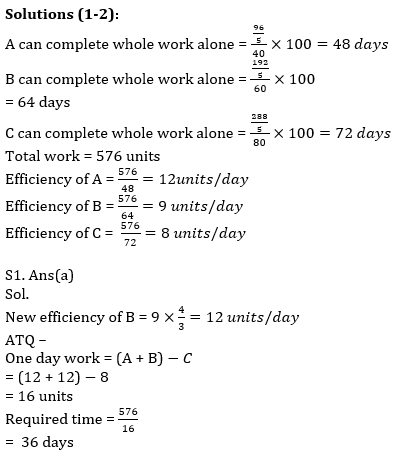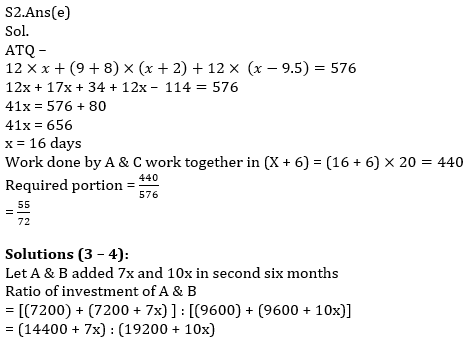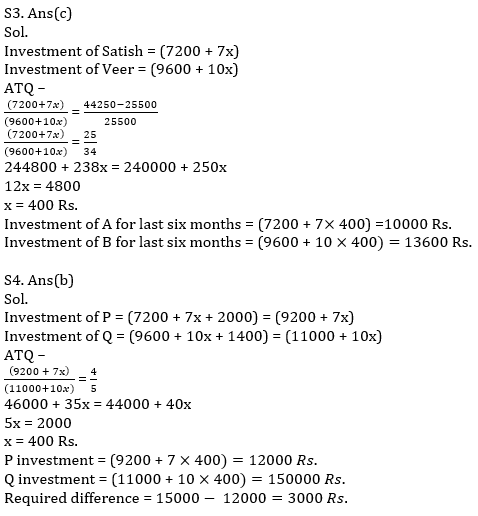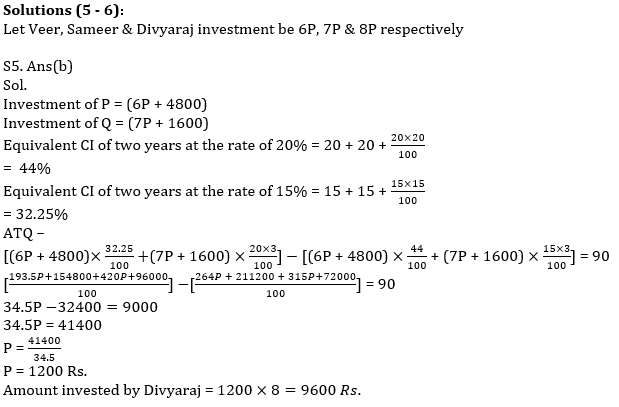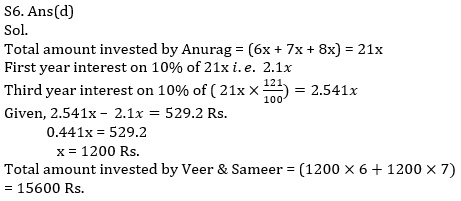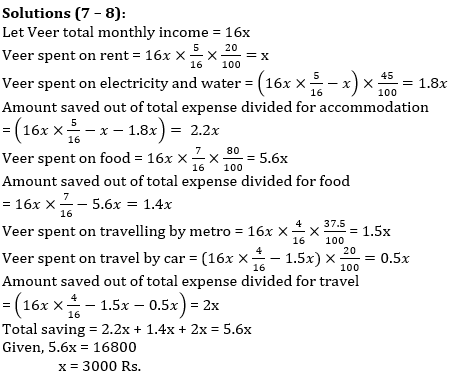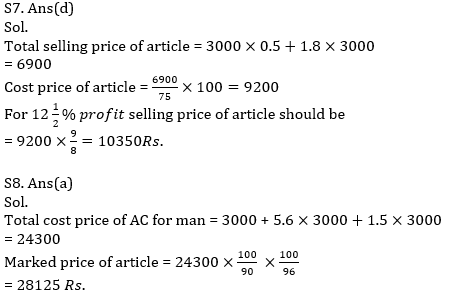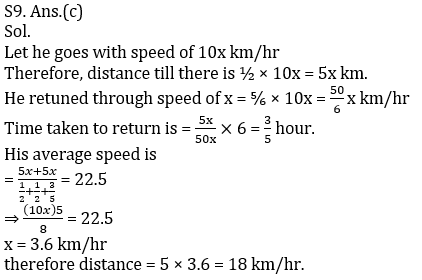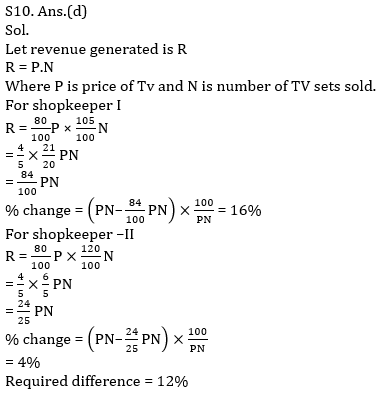Click Here to Register for Bank Exams 2021 Preparation Material#### Congratulations!Download Hindu Review of October 2021: Free PDF